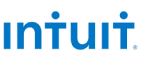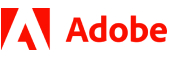New update is available. Click here to update.
Topics

# Max Game

Easy0/40
Average time to solve is 25m+2 more companies

## Problem statement

You are given 'N' numbers and you have to play a game using them. In one move you have to pick any two numbers 'A' and 'B' and replace them by their sum 'A+B'. Doing this gives you a penalty of 'A+B'. Note that the count of elements reduces by 1 every time you take 2 numbers and replace them by their sum. The game ends when there is only one element left. Your task is to minimise the penalty during the game.

You need to return the minimum possible penalty you can have when the game ends.

Detailed explanation ( Input/output format, Notes, Images )
Constraints:
``````1 <= T <= 5
1 <= N <= 10^5
1 <= X[i] <= 10^6

Time limit: 1 second
``````
Sample input 1:
``````1
4
2 3 1 4
``````
Sample output 1:
``````19
``````
Explanation
``````To achieve the minimum penalty,
First, we pick 1 and 2, replace it by 3. We get { 3, 3, 4}
Second, we pick 3 and 3, replace it by 6. We get {4, 6}
Third, we pick 4 and 6, replace it by 10. We get {10}

The minimum penalty will be 3 + 6 + 10 = 19.
``````
Sample input 2:
``````1
5
7 6 5 5 3
``````
Sample output 2:
``````60
``````Console